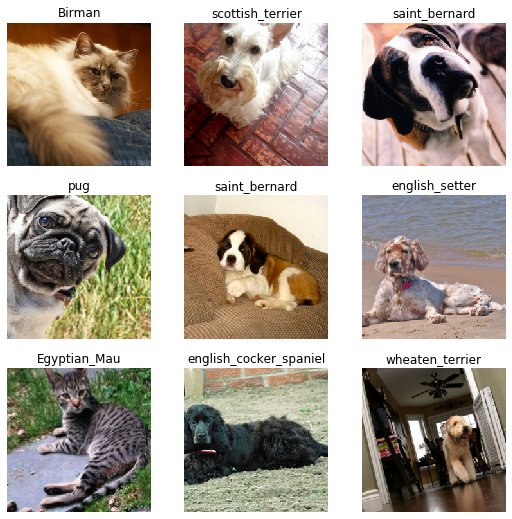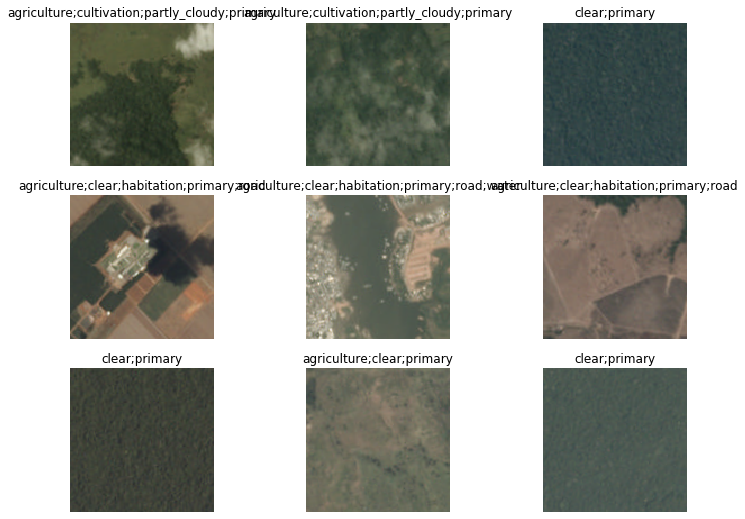Fine-tuning a pretrained model on the pets dataset.
from fastai2.basics import *
from fastai2.callback.all import *
from fastai2.vision.all import *

# all_slow


## Single-label classification¶

### Gathering the data¶

We use the data block API to get our data in a DataLoaders. Here our inputs are images and our targets categories. The images are all in a folder, so we use get_image_files to collect them all, a RandomSplitter to split between training and validation, then we get the label from the filenames with a regex labeller.

pets = DataBlock(blocks=(ImageBlock, CategoryBlock),
get_items=get_image_files,
splitter=RandomSplitter(),
get_y=RegexLabeller(pat = r'/([^/]+)_\d+.jpg\$'),
item_tfms=Resize(460),
batch_tfms=aug_transforms(size=224, max_rotate=30, min_scale=0.75))


The pets object by itself is empty: it only containes the functions that will help us gather the data. We have to call its datasets or dataloaders method to get a Datasets or a DataLoaders. The first thing we need to pass to either of those functions is the source, here the folder where all the images are. Then we specify some dataset transforms (a random resized crop to 300 by 300) and some dataloader transforms (basic data augmentation on the GPU and normalization using the imagenet statistics).

dls = pets.dataloaders(untar_data(URLs.PETS)/"images",  bs=32)


Then we can look at some of our pictures with dls.show_batch()

len(dls.train_ds.items)

5912
dls.show_batch(max_n=9)### Using a pretrained model¶

First let's import a resnet34 form torchvision.

from torchvision.models import resnet34,resnet50

#from fastai2.vision.models.xresnet import xresnet50


opt_func = partial(Adam, lr=slice(3e-3), wd=0.01, eps=1e-8)

#Or use Ranger


Then we can call cnn_learner to build a Learner from our DataLoaders. Since we are using a pretrained model, it comes automatically frozen, which means only the head is going to be trained.

learn = cnn_learner(dls, resnet50, opt_func=opt_func, metrics=error_rate, config=cnn_config(ps=0.33)).to_fp16()


We can train the head a little bit using the 1cycle policy.

learn.fit_one_cycle(1)

epoch train_loss valid_loss error_rate time
0 0.539979 0.260939 0.084574 00:24

Then we can unfreeze the model and use discriminative learning rates.

learn.unfreeze()
# learn.fit_one_cycle(4, slice(1e-5, 1e-3))
learn.fit_one_cycle(1, slice(1e-5, 1e-3))

epoch train_loss valid_loss error_rate time
0 0.480262 0.255037 0.082544 00:31

### Seeing results¶

fname = dls.train_ds.items

learn.predict(fname)

('Russian_Blue',
tensor(9),
tensor([3.2060e-04, 4.6202e-06, 1.6596e-04, 9.3935e-02, 1.7009e-01, 2.0974e-04,
2.1056e-04, 4.9679e-04, 6.3355e-04, 7.3025e-01, 4.8957e-04, 3.2960e-05,
9.3550e-05, 4.3036e-04, 9.2063e-06, 2.8626e-04, 6.1277e-05, 9.9583e-05,
1.1156e-04, 5.2107e-05, 3.9530e-04, 1.6063e-05, 1.0351e-05, 1.0120e-04,
1.2156e-04, 1.9338e-05, 8.3837e-05, 5.4829e-04, 1.4427e-05, 1.2248e-04,
4.9762e-06, 1.0287e-04, 7.7992e-05, 6.1068e-05, 1.0534e-04, 1.7529e-04,
5.1625e-05]))
learn.export()

img = PILImage.create(fname)

learn1.show_results(max_n=9)

interp = Interpretation.from_learner(learn1)

interp.plot_top_losses(9, figsize=(15,10))


## Multi-label classification¶

planet_source = untar_data(URLs.PLANET_TINY)

planet = DataBlock(blocks=(ImageBlock, MultiCategoryBlock),
get_x=lambda x:planet_source/"train"/f'{x}.jpg',
splitter=RandomSplitter(),
get_y=lambda x:x.split(' '),
batch_tfms=aug_transforms(flip_vert=True, max_lighting=0.1, max_zoom=1.05, max_warp=0.))

dls.show_batch(max_n=9, figsize=(12,9))learn = cnn_learner(dls, resnet34, metrics=accuracy_multi)
learn.fit_one_cycle(1)

epoch train_loss valid_loss accuracy_multi time
0 1.103069 0.649033 0.610714 00:02
learn.predict(planet_source/f'train/train_10030.jpg')

((#5) ['bare_ground','clear','cloudy','cultivation','road'],
tensor([False, False,  True, False,  True,  True,  True, False, False, False,
False,  True, False, False]),
tensor([0.4993, 0.2256, 0.6531, 0.3821, 0.7002, 0.6430, 0.6785, 0.4854, 0.2270,
0.4699, 0.4722, 0.7407, 0.1130, 0.1830]))
learn.tta(use_max=True)

img = PILImage.create(planet_source/f'train/train_10030.jpg')

learn.predict(img)

learn.show_results(max_n=9)

interp = Interpretation.from_learner(learn)

interp.plot_top_losses(9)


## Segmentation¶

codes = np.loadtxt(untar_data(URLs.CAMVID_TINY)/'codes.txt', dtype=str)
def get_y(o): return path/'labels'/f'{o.stem}_P{o.suffix}'

path = untar_data(URLs.CAMVID_TINY)

dls.show_batch(max_n=4)